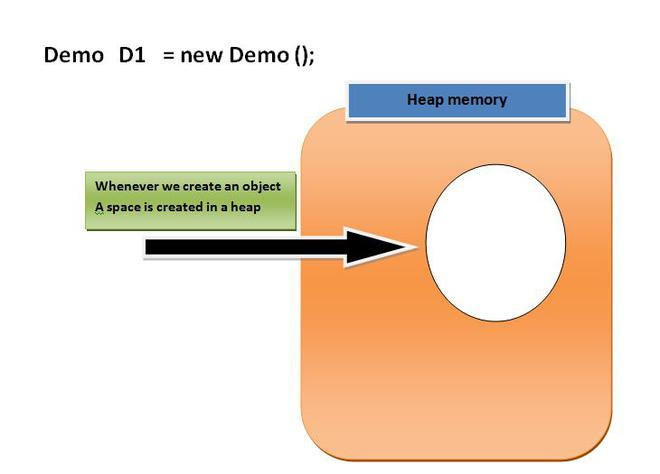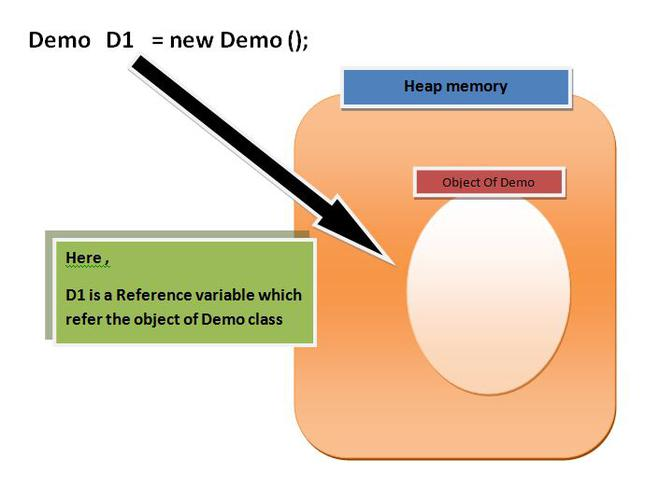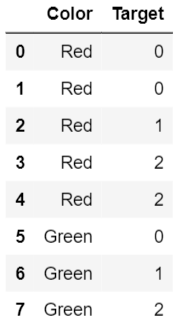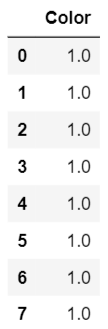1594696200

# What are categorical variables and how to encode them?

Introduction

In this article, we will explain what categorical variables are and we will learn the difference between different types of them. We will discuss:

nominal categorical variables

versus

ordinal categorical variables.

Finally, we will learn what are the best methods for encoding each categorical variable type with examples. We will cover:

One hot encoding

and

integer encoding.

What are categorical variables?

In order to understand categorical variables, it is better to start with defining continuous variables first. Continuous variables can take any number of values. A good example of the continuous variable is weight or height. They both can take theoretically any value.

Categorical variables are variables in the data set that unlike continuous variables take a finite set of values. For example, grades that students are given by a teacher for assignments (A, B, C, D, E, and F).

Another example of a categorical variable is jersey color that a college is selling. Imagine that they are selling it only in green, blue, and black. Jersey color would be a categorical variable with three possible values.

In the dataset, categorical variables are often strings. In the two examples, we have seen above, they are strings as both grades and color values had this data type.

However, we need to be careful as sometimes integers could hide categorical as well. Therefore it is important to see how many unique values an integer variable has before deciding if it is a continuous or a categorical variable.

Nominal categorical variables

The examples of categorical variables that we have been given above are not identical. There is a difference between jersey color and grades as your intuition may suggest. The colors of the jersey that were green, blue, and black do not have any kind of ordering between themselves. If there is a lack of any kind of logical ordering between the values of the categorical variable we call it a nominal variable.

#machine-learning #technology #data-science #data analysis

## Buddha Community1623967440

## Reference Variable in Java

Before We Started with the Reference variable we should know about the following facts.

**1. **When we create an object(instance) of class then space is reserved in heap memory. Let’s understand with the help of an example.

Demo D1 = new Demo();Now, The space in the heap Memory is created but the question is how to access that space?.

Then, We create a Pointing element or simply called Reference variable which simply points out the Object(the created space in a Heap Memory).**Understanding Reference variable **

1. Reference variable is used to point object/values.

**2. **Classes, interfaces, arrays, enumerations, and, annotations are reference types in Java. Reference variables hold the objects/values of reference types in Java.

3. Reference variable can also store null value. By default, if no object is passed to a reference variable then it will store a null value.

**4. **You can access object members using a reference variable using **dot **syntax.

.<instance variable_name / method_name>

#java #reference variable in java #variables in java #reference variables #refer #variable's1623384600

## Scope of a Variable In Java [With Coding Example]

### Introduction

Programmers define the scope of a Variable in Java that tells the compiler about the region from where a variable is accessible or visible. The scope of a variable in Java is static by nature. It means we have to declare it at compile time only. In this article, you will learn about the scope of a Java variable along with its types

### Conclusion

#full stack development #java variable #scope of a variable in java #variable #scope of a variable #scope1598524800

## Target Encoding For Multi-Class Classification

This article is in continuation of my previous article that explained how target encoding actually works. The article explained the encoding method on a binary classification task through theory and an example, and how category-encoders library gives incorrect results for multi-class target. This article shows when _TargetEncoder of category_encoders fails, gives a snip of the theory behind encoding multi-class target, _and provides the correct code, along with an example.

### When does the TargetEncoder fail?

Look at this data. Color is a feature, and Target is well… target. Our aim is to encode Color based on Target.Let’s do the usual target encoding on this.

``````import category_encoders as ce

ce.TargetEncoder(smoothing=0).fit_transform(df.Color,df.Target)
``````Hmm, that doesn’t look right, does it? All the colors were replaced with 1. Why? Because TargetEncoder takes mean of all the Target values for each color, instead of probability.

#multi-class #categorical-variable #categorical-data #target-encoding #multiclass-classification #big data1594282200

## Unleash the Power of Pandas ‘category’ Dtype: Encode Categorical Data in a Smarter Way

Tutorials on using Pandas’ category’ data type in Python

#pandas #unleash #power #encode #categorical #smarter1594815386

## HTML URL Encoding

Uniform Resource Locator or URL is used as the address of a document on the web. It can be composed of words, typically the Domain Name Server(DNS), or IP address. For example, https://data-flair.training/blogs/ is a URL.

The structure of this URL is as follows-

scheme://prefix.domain:port/path/filename

Here:

Scheme – Defines the internet service type, commonly http or https.

Prefix – Defines the domain prefix, www.

Domain – It defines the domain name of the internet, data-flair.training

Port – Defines the host’s port number, 80 is the default port number for http.

Path – Defines the path at the server.

Filename – It defines the name of the file or the document that is being displayed.

## HTML URL Schemes

Some common URL schemes are-

• Http(HyperText Transfer Protocol) – Used for common web-pages. It is not encrypted.
• Https(Secure HyperText Transfer Protocol) – Used for secure web-pages. It is encrypted.